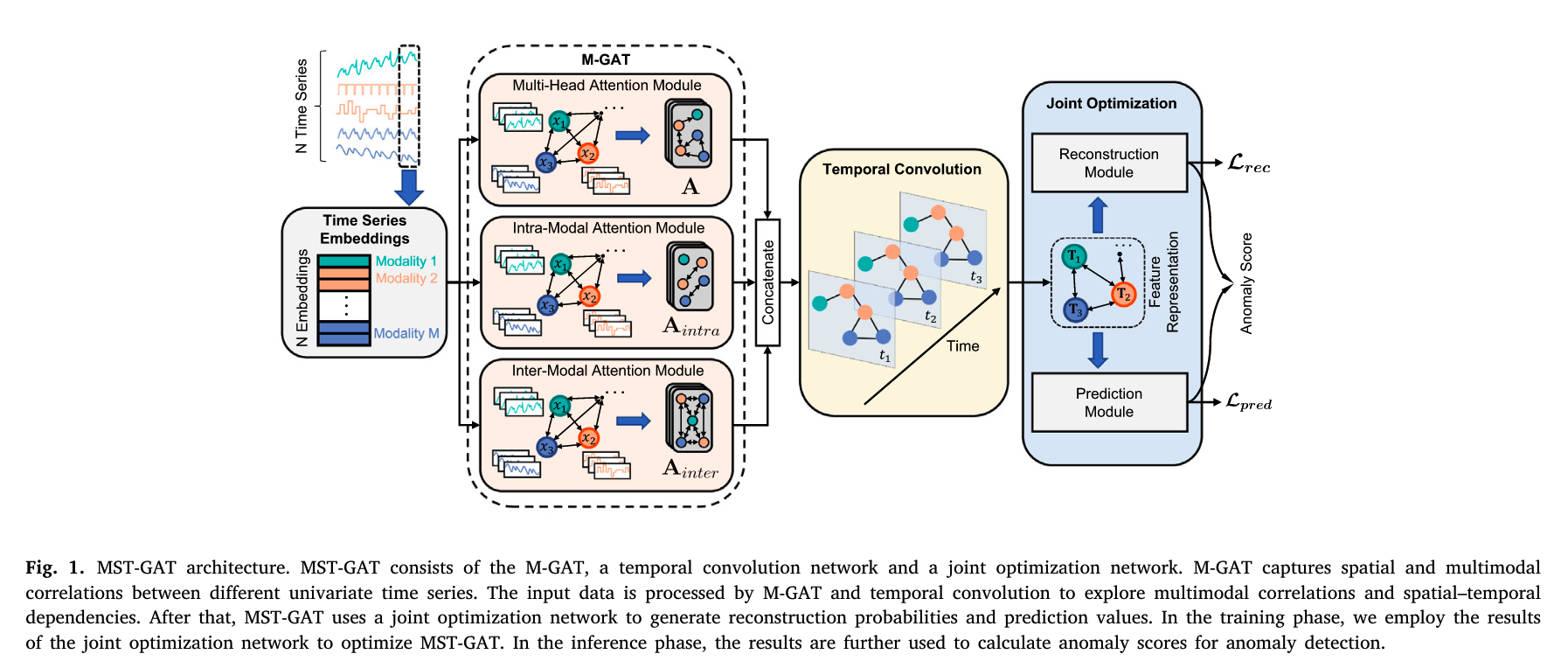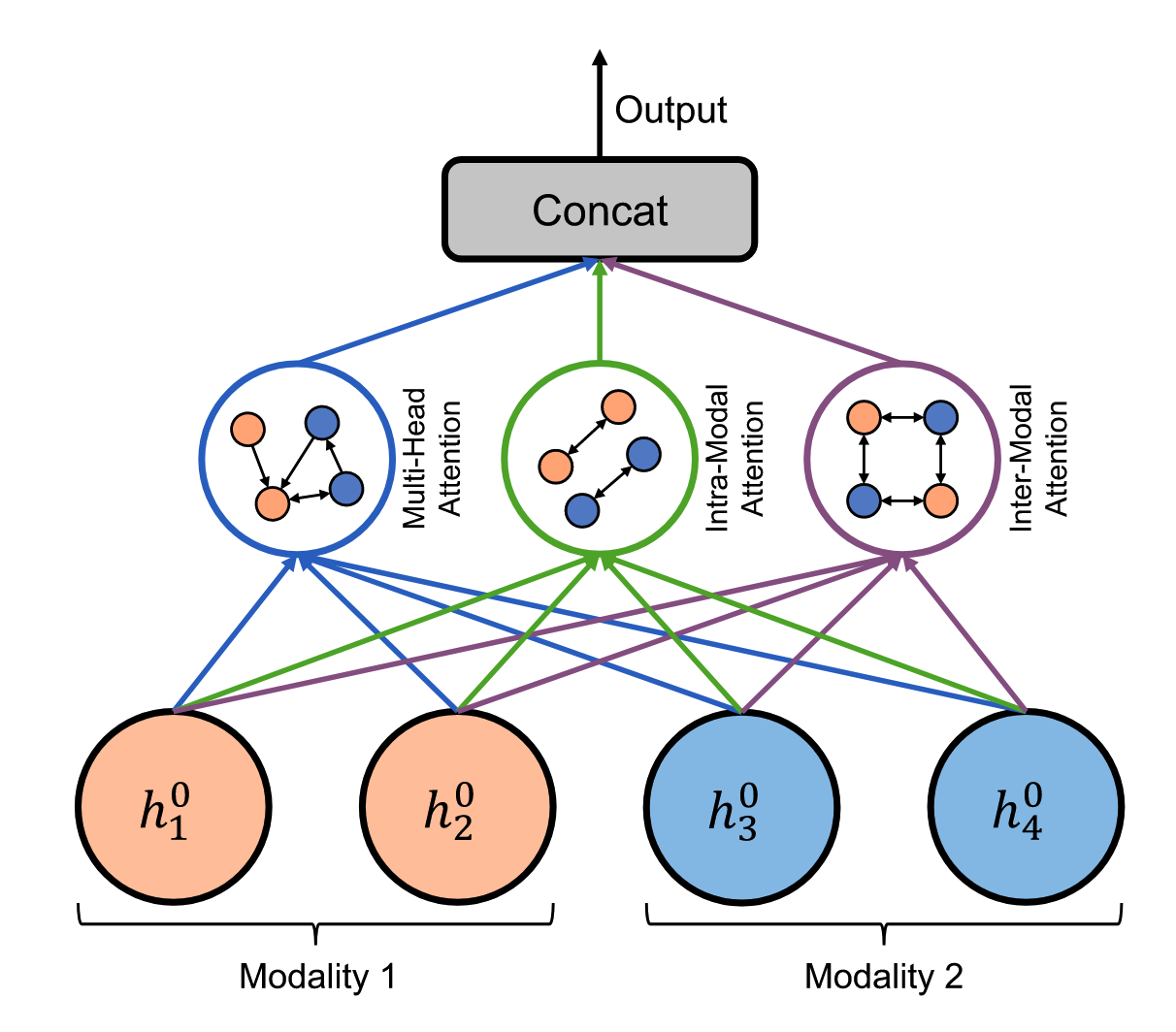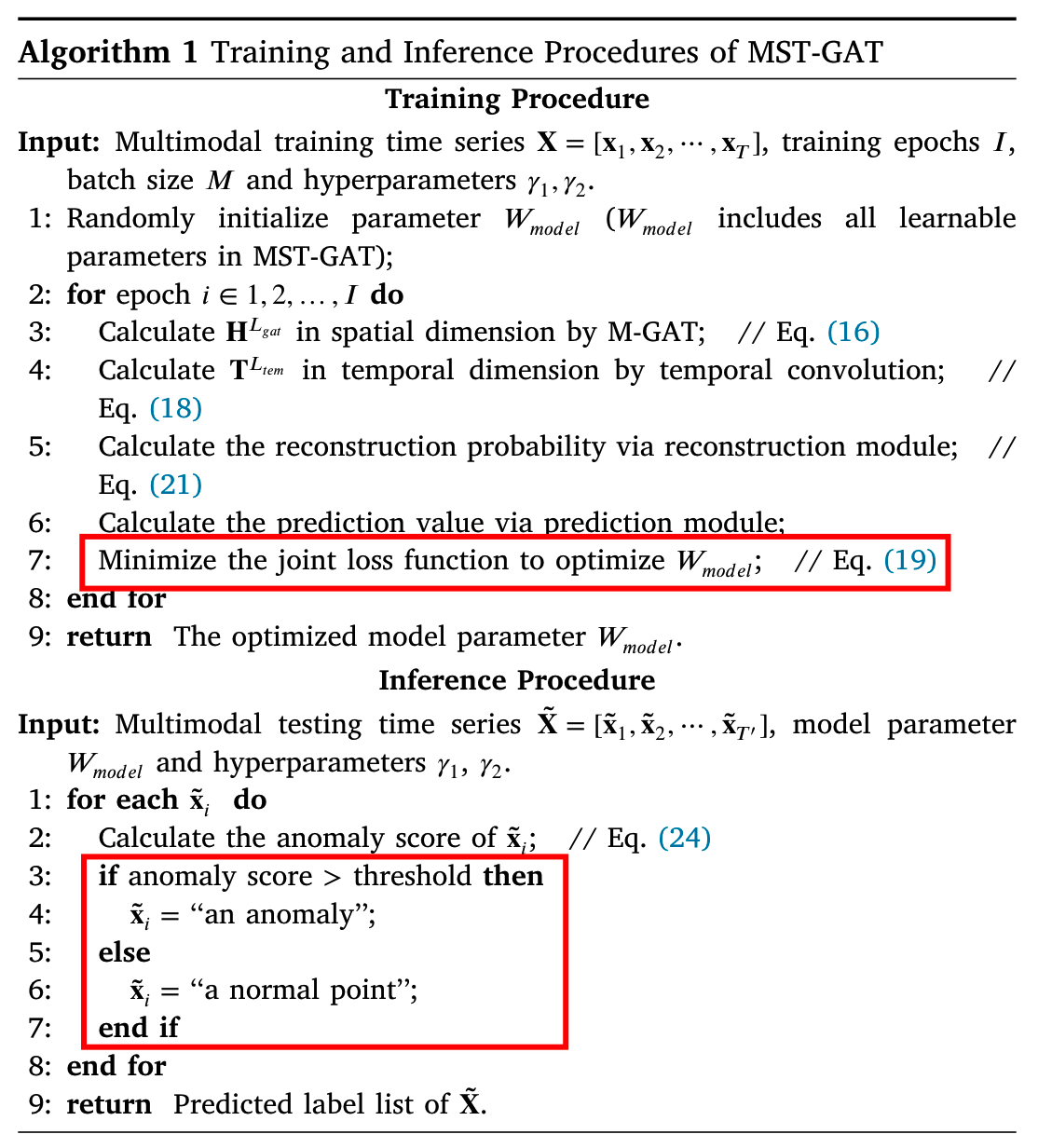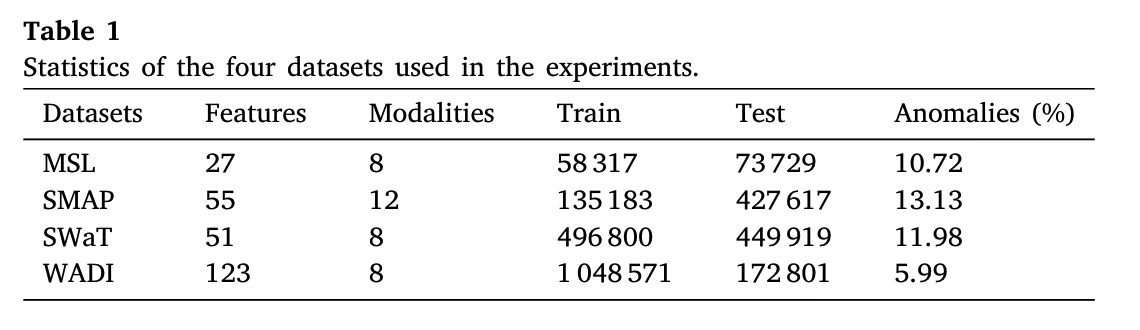• 累计撰写 57 篇文章
• 累计创建 34 个标签
• 累计收到 7 条评论

### 目 录CONTENT# 阅读笔记MST-GAT: A multimodal spatial–temporal graph attention network for time series anomaly detection2023-09-04 / 0 评论 / 0 点赞 / 13 阅读 / 5401 字

# 7.28 MST-GAT: A multimodal spatial–temporal graph attention network for time series anomaly detection

Source: Information Fusion 一些缩写： MTS: multimodal time series

## 动机

1. 单一的单变量时间序列无法反应实体的整体状态，简单地将多个单变量时间序列的检测结果结合起来的方法往往表现不佳
2. 【数据和特征捕获方面的问题】以往的MTS方法考虑了时间依赖性，能捕获到时间维度上的动态变化，但是忽略了不同时间序列之间的空间依赖性，尽管有些改进，但是仍没能显式地捕捉多模态时间序列之间的多模态相关性
3. 预测的可解释性问题（8.15 Towards Graph-level Anomaly Detection via Deep Evolutionary Mapping 这篇文章也提到了该问题）也许异常的检测结果的可解释性问题很重要

### 用了什么网络

1. multimodal 用于明确捕捉多模态时间序列之间的模态依赖关系和空间依赖关系：
2. 两个关系注意力模块 relational attention module
1. intra-modal attention 内部modal
2. inter-modal attention 外部modal
2. 时序卷积网络 temporal convolutional network，用于捕获每个时间序列中的时间依赖关系 temporal dependency

### 其他模块

1. 重构模块：用于重构输入数据。它帮助网络学习对原始数据进行重构，以保留重要信息和特征，有助于提高整体的数据表征能力。
2. 预测模块：用于预测下一个时间戳的特征。通过预测未来的特征，网络可以理解数据的演进趋势和可能出现的异常情况。

## MST-GAT方法概览1. 网络结构学习 Graph structrue learning “to learn a graph structure in the spatial dimension”
2. Multimodal Graph Attention Network (M-GAT) 它包含三个注意力模块
1. 多头注意力
2. 两个关系注意力
3. 时序卷积网络
4. 联合优化 “optimizes both reconstruction and prediction targets”

## 方法细节

1. 数据格式&问题定义（有公式，见notability）
2. 问题定义： $X \rightarrow y$ ，y是序列，有 $T^\prime$ 个元素，每个元素都是1/0，表示有无异常
3. 图结构学习：
1. 面向的是time series，先认为初始的embedding $v_i$ 是已经通过默写方法获得了，接下来的首要目标是通过这些向量构造出一个图网络
2. 衡量节点相似度（cosine similarity），对每个节点选出top k个最大相似度的节点，然后构造矩阵A（节点j在节点i的top k里面，则$A_{ij} = 1$ ，否则=0）。于是邻接矩阵A能够北构造出，进而有了图网络
4. 空间维度？滑动窗口，每次从X中观测w个x对于训练时间序列X，使用长度为w的滑动窗口在每个时刻产生一个固定长度的输入。定义 X为t时刻M-GAT的输入 M-GAT由三个注意模块组成，即多头注意、通道内注意和通道间注意。多头注意力模块侧重于建模多模态时间序列之间模态无关的空间关系，而模态内和模态间注意力模块侧重于捕捉不同时间序列之间的多模态相关性。
5. 时序维度卷积。多模态图注意力在空间维度上捕获每个节点的邻居信息，而时间卷积网络在时间维度上应用立场卷积来捕获时间动态。
6. 联合优化：两个任务：reconstruction和prediction，loss函数包含两部分优化目标 $$\mathcal{L}=\gamma_1 \mathcal{L}{\text {rec }}+\left(1-\gamma_1\right) \times \mathcal{L}{\text {pred }}$$ 前面那个loss是reconstruction的，后面的是prediction模块的
1. reconstruction module：该模块的目标是学习输入数据的重构概率，使用VAE (Variational autoencoder) 去重构图网络，给定输$X_t$，VAE用条件概率$p_\psi(\mathcal{X}t|z_t)$来重构X，z是representation。训练重构模块的目标是最大化zt的后验分布： $$p\psi(z_t|\mathcal{X}t)=p\psi(\mathcal{X}t|z_t)p\psi(z_t)/p_\psi(\mathcal{X}t)$$ 最后一项可以给出形式化定义但是很难计算，所以用另一个模型去近似它，最后，修改后的重构损失为： \begin{aligned}\mathcal{L}{rec}&=-\mathrm{E}{q\rho(z_t|\mathcal{X}t)}[logp_\psi(\mathcal{X}_t|z_t)]\&+D{KL}(q_\rho(z_t|\mathcal{X}t)\parallel p\psi(z_t))\end{aligned}
2. prediction module：用MLP作为时序卷积后的预测网络 $$\mathcal{L}{pred}=\frac1{T-w}\sqrt{\sum{i=1}^N(x_{i,t+1}-\hat{x}_{i,t+1})^2}$$
3. 异常打分、推断：上面两个模块分别生成重构概率$p_i$和预测$\hat{\bf{x}}{i}$，每个时间戳的最终异常分数是每个时间序列的异常分数之和 $$\mathrm{score}=\sum{i=1}^N\frac{(1-p_i)+\gamma_2\times(\mathbf{x}_i-\hat{\mathbf{x}}_i)^2}{1+\gamma_2}$$ 推断阶段对评分通过设定阈值的方法判断每个时间步上是异常或非异常，阈值如何选定——peaks-over-threshold (POT)算法## 实验

### 数据集### baselines

#### 单模块的

PCA: Principal component analysis

AE: Autoencoder

DAGMM: Deep autoencoding Gaussian model

LSTM-VAE: LSTM-VAE substitutes the fully connected network in variational autoencoder with LSTM

#### 多模块的

OmniAnomaly: OmniAnomaly

GDN: Graph deviation network

## 感悟

1. 本文的研究对象本身虽然不是图，但是它最后检测出来的东西依然是图级别的
2. 并非要能够直接建模成图网络的东西才能够用图异常检测技术。如果能够通过某些方式获得样本的向量，通过很多方法如相似度计算，就能够人为的构造出一个网络，基于这个网络就能够做异常检测的许多工作，包括节点、边、图等等
0
• \${post.likes!0}